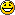Tabla de Contenidos

## Total Probability

We have the followings n eventsthat are:

• they are incompatible events two by two, ie, they haven´t common elements ()

• if we join all events from E (colection of samples), is the group that is created by all posible results from a random experience.

That:Then, the probability of a event S is happened, I can decompose it, as:(Demostration of this result)

### Example

A mouse is persecuted by a hungry cat. The mouse can to choose one of these streets A, B or C, to salve himself.

• The probability that the mouse choose the A street is 0.3P(A)=0.3
• The probability that the mouse choose the B street is 0.5P(B)=0.5
• The probability that the mouse choose the C street is 0.2P(C)=0.2

The probabilities that the mouse is hunted by mouse in every street, are:

• P(cat hunts mouse in A)= P(+|A)= 0.4
• P(cat hunts mouse in B)= P(+|B)= 0.6
• P(cat hunts mouse in C)= P(+|C)= 0.1What´s the probability that cat hunts mouse?.(Calculate P(+))

SOLUTION

We make a diagram with tree form to this exercise:P(+)==¡the cat does not have assured the food !total_probability.txt · Última modificación: 24/04/2017 13:13 (editor externo)